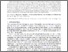Open access research
publication repository

# Fast Random Integer Generation in an Interval [r-libre/1437]

Lemire, Daniel (2019). Fast Random Integer Generation in an Interval. ACM Transactions on Modeling and Computer Simulation, 29 (1). https://doi.org/10.1145/3230636

File(s) available for this item:PDF - rangedrng.pdf Content : Submitted Version License : Creative Commons Attribution. Download
 Item Type: Journal Articles Yes Published In simulations, probabilistic algorithms and statistical tests, we often generate random integers in an interval (e.g., [0,s)). For example, random integers in an interval are essential to the Fisher-Yates random shuffle. Consequently, popular languages like Java, Python, C++, Swift and Go include ranged random integer generation functions as part of their runtime libraries. Pseudo-random values are usually generated in words of a fixed number of bits (e.g., 32 bits, 64 bits) using algorithms such as a linear congruential generator. We need functions to convert such random words to random integers in an interval ([0,s)) without introducing statistical biases. The standard functions in programming languages such as Java involve integer divisions. Unfortunately, division instructions are relatively expensive. We review an unbiased function to generate ranged integers from a source of random words that avoids integer divisions with high probability. To establish the practical usefulness of the approach, we show that this algorithm can multiply the speed of unbiased random shuffling on x64 processors. Our proposed approach has been adopted by the Go language for its implementation of the shuffle function. Lemire, Daniel Daniel Lemire 28 May 2018 15:19 15 Dec 2021 19:18RÉVISER